A 5.00-kg box slides 4.00 m across the floor before coming to rest. What is the coefficient of kinetic friction between the floor and the bo

Question

A 5.00-kg box slides 4.00 m across the floor before coming to rest. What is the coefficient of kinetic friction between the floor and the box if the box had an initial speed of 3.00 m/s?

in progress 0
5 months 2021-08-28T07:56:18+00:00 1 Answers 2 views 0

1. Explanation:

According to the given situation,

work done = change in kinetic energy

As the formula of kinetic energy is as follows.

K.E =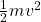Putting the given values into the above formula as follows.

K.E ==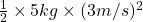= 22.5 J

Also we know that,

Work done = force x distance

or,          force =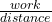=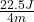= 5.62 N

In the given case, force is friction and formula to calculate friction is as follows.

friction =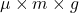where,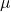= the coefficient of friction

Putting the given values into the above formula as follows.

Friction =5.62N =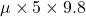= 0.114

Thus, we can conclude that the coefficient of kinetic friction between the floor and the given box is 0.114.